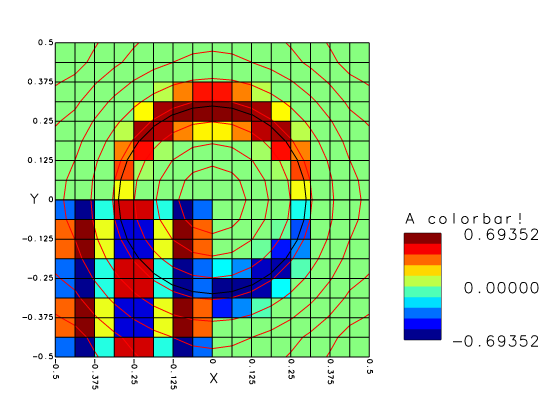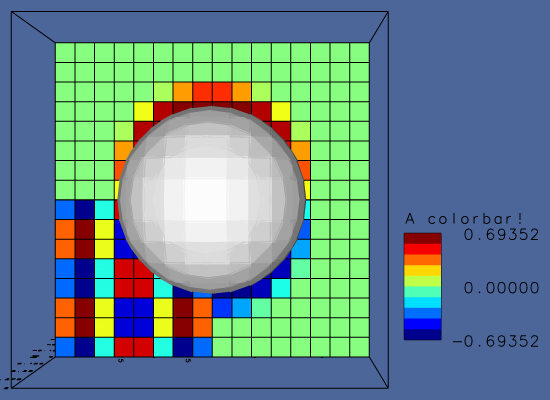# Simple test of Basilisk View

Ran in parallel on four MPI processes.

#include "fractions.h"
#include "view.h"

int main() {

We first define a volume fraction field.

  init_grid (16);
origin (-0.5,-0.5,-0.5);
scalar f[];
fraction (f, sq(x) + sq(y) + sq(z) - sq(0.3));

Then display it using Basilisk view functions.2D Basilisk view 3D Basilisk view
  view (width = 400, height = 400, cache = 10);
box();
draw_vof ("f");
cells();
squares ("x < 0 && y < 0 ? sin(6*pi*x)*cos(8*pi*y) : nodata", spread = -1);
squares ("(f[0,1] - f[0,-1])/(2.*Delta)", spread = -1);
#if dimension == 2
isoline ("sqrt(x^2 + y^2)", n = 10, spread = -1, lc = {1,0,0});
#endif
save ("out.png");

A few more files just for testing.

  output_facets (f, qerr);
dump (file = "dump");
}